# The cantilevered beam shown here has a known rigidity, EI, and length, b, and is loaded...

The cantilevered beam shown here has a known rigidity, EI, and length, b, and is loaded with a point force and a point moment as shown.

(a) Determine all reactions forces and draw the shear and moment diagrams for this loading.

(b) Using discontinuity functions and the integration method, find the deflection and the slope of the beam at the free end.

(c) Using the moment-area method, find the deflection and the slope of the beam at the location of the point load.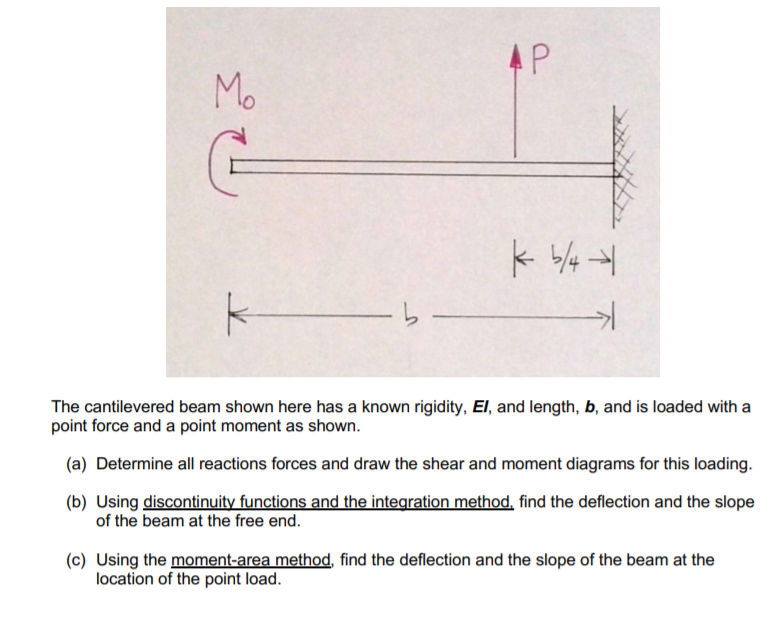AP Mo G K 6/4 -> k The cantilevered beam shown here has a known rigidity, El, and length, b, and is loaded with a point force and a point moment as shown. (a) Determine all reactions forces and draw the shear and moment diagrams for this loading. (b) Using discontinuity functions and the integration method, find the deflection and the slope of the beam at the free end. (c) Using the moment-area method, find the deflection and the slope of the beam at the location of the point load.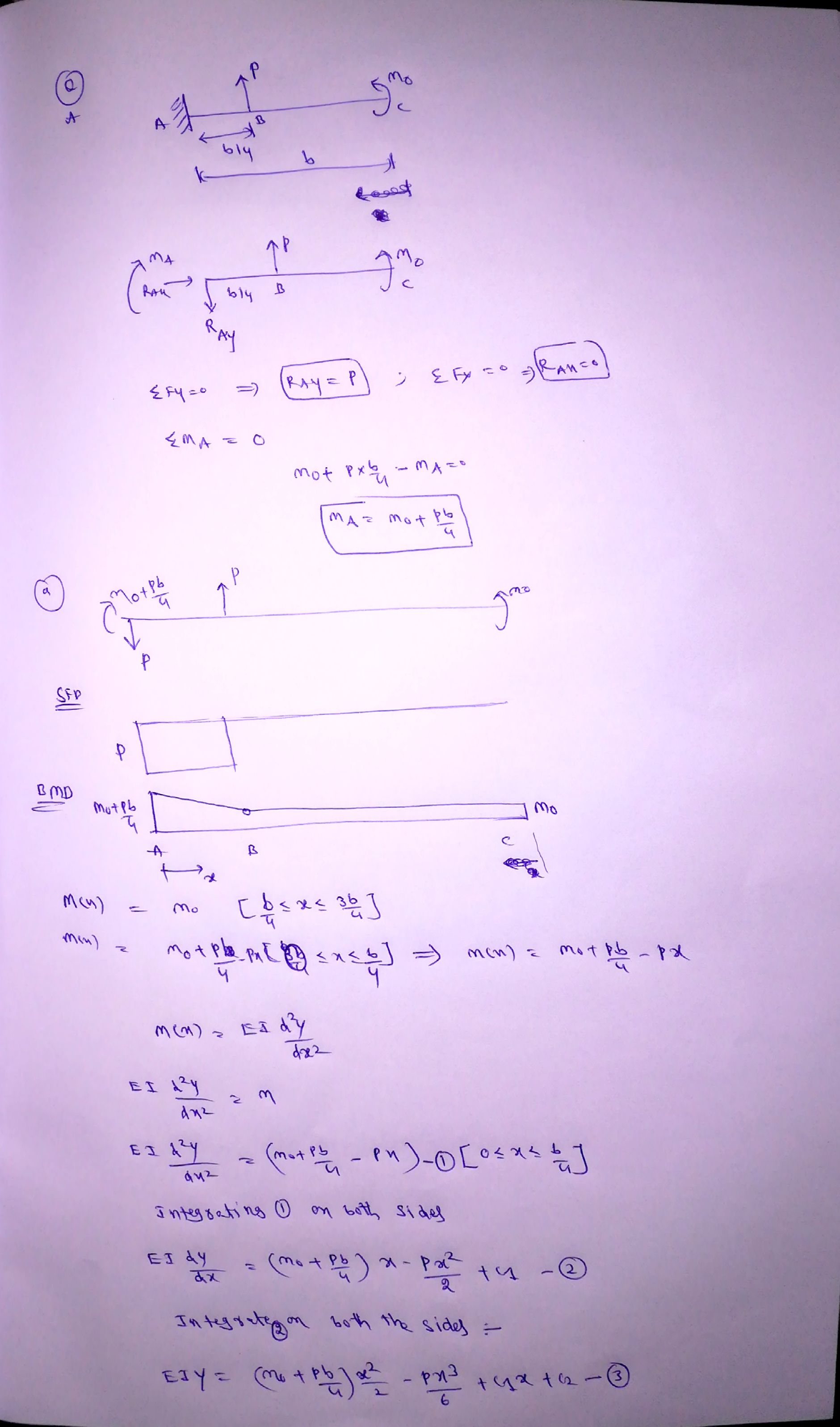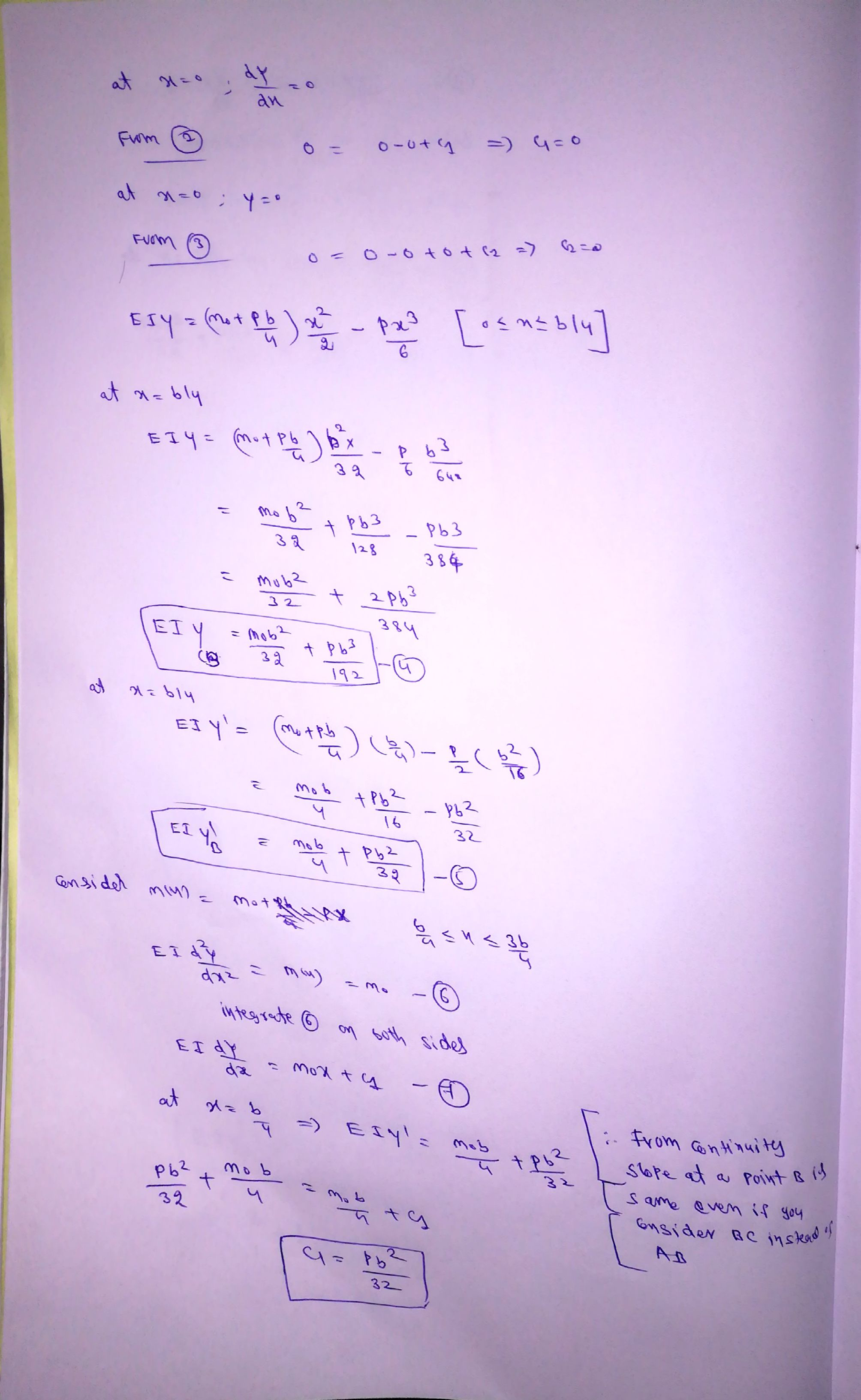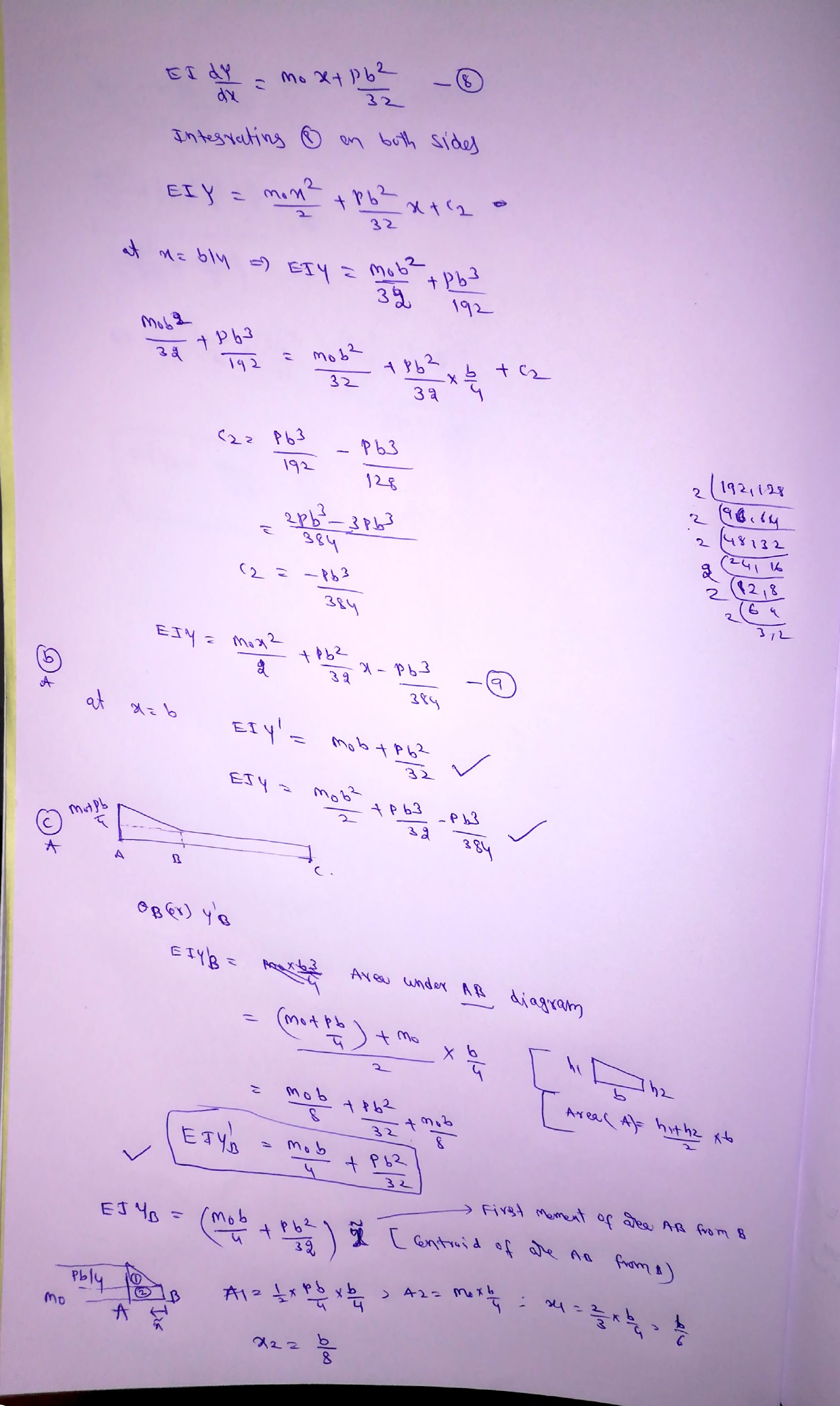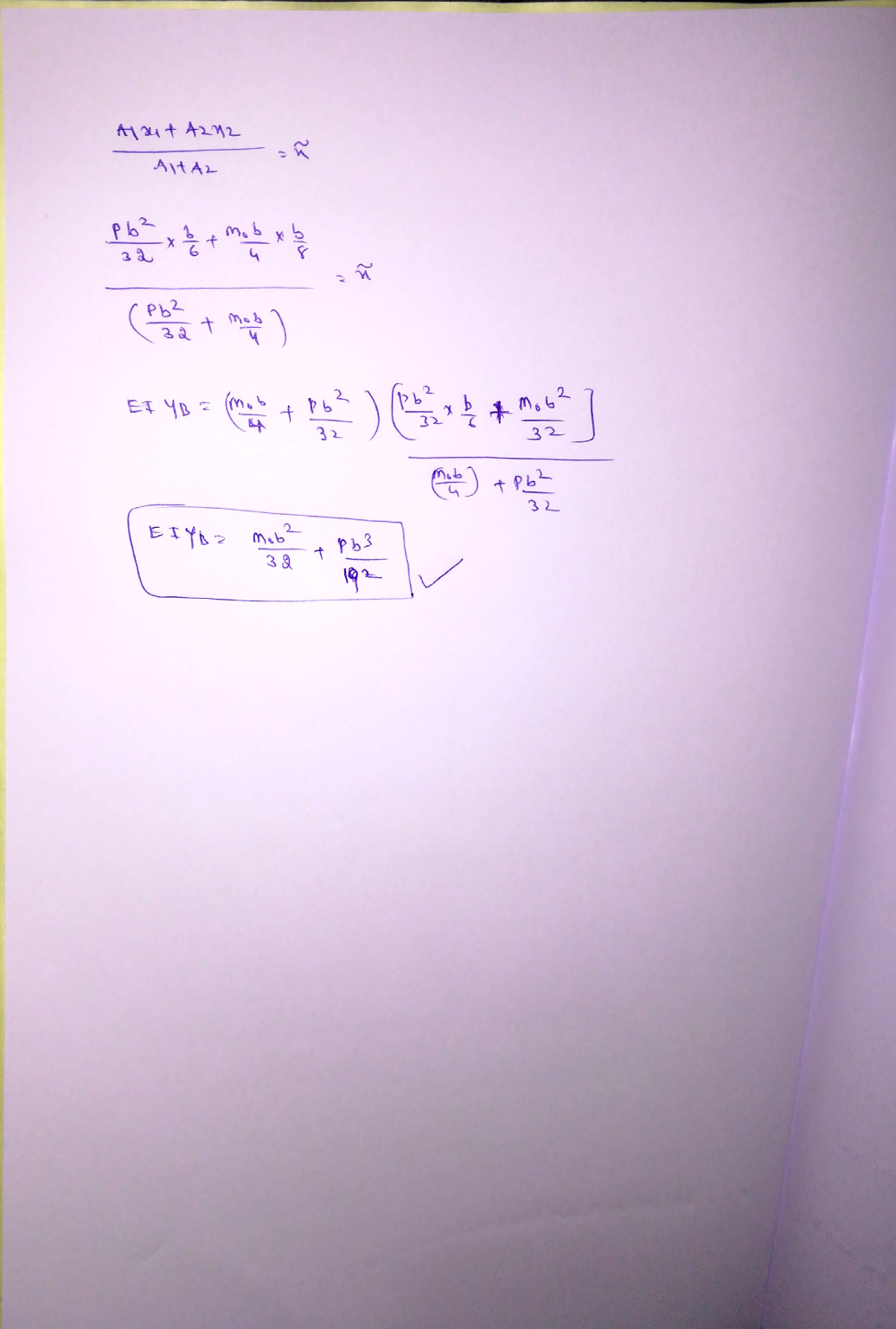#### Earn Coin

Coins can be redeemed for fabulous gifts.

Similar Homework Help Questions
• ### The cantilevered beam shown here has a known rigidity, EI, and length, b, and is loaded...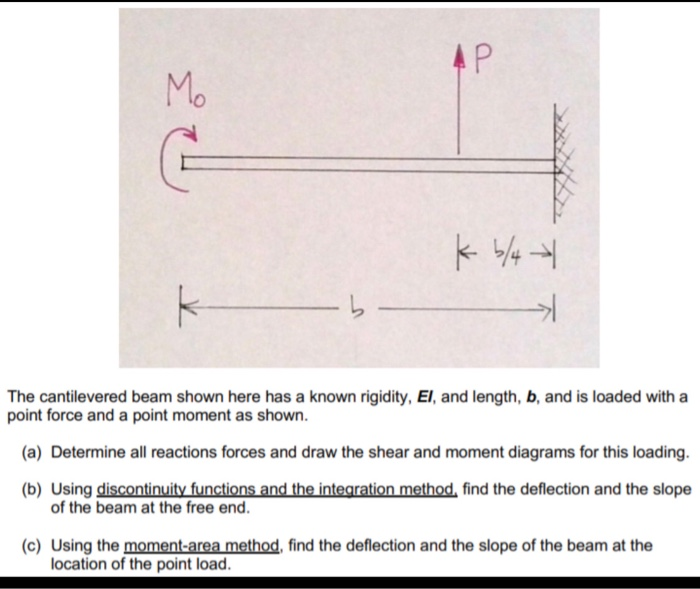The cantilevered beam shown here has a known rigidity, EI, and length, b, and is loaded with a point force and a point moment as shown a) Determine all reactions forces and draw the shear and moment diagrams for this loading. b) Using discontinuity functions and the integration method, find the deflection and the slope of the beam at the free end. c) Using the moment-area method, find the deflection and the slope of the beam at the location of...

• ### AP Mo k -> The cantilevered beam shown here has a known rigidity, El, and length,...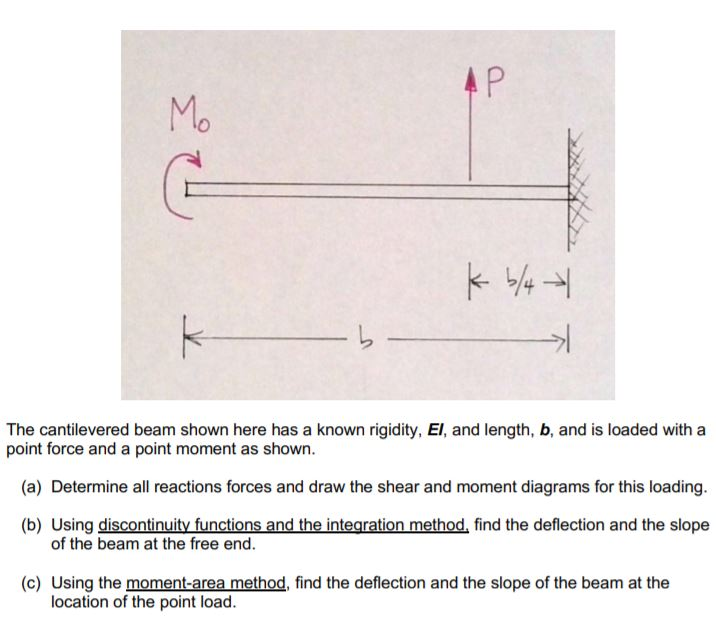AP Mo k -> The cantilevered beam shown here has a known rigidity, El, and length, b, and is loaded with a point force and a point moment as shown. (a) Determine all reactions forces and draw the shear and moment diagrams for this loading. (b) Using discontinuity functions and the integration method, find the deflection and the slope of the beam at the free end. (c) Using the moment-area method, find the deflection and the slope of the beam...

• ### AP Mo kb/4 K The cantilevered beam shown here has a known rigidity, El, and length,...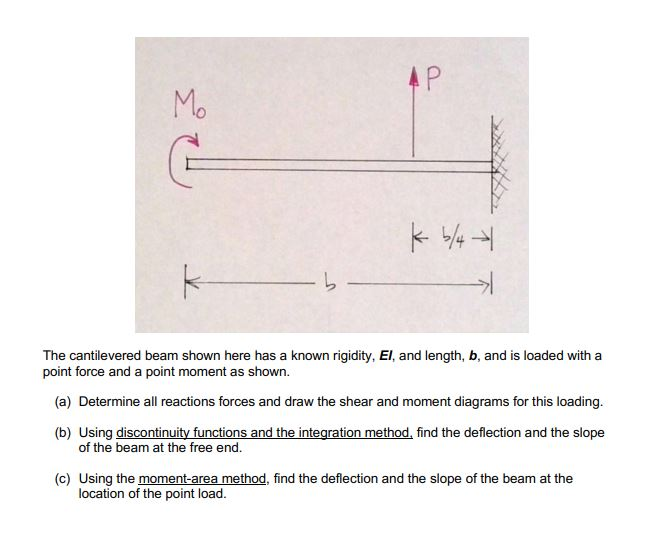AP Mo kb/4 K The cantilevered beam shown here has a known rigidity, El, and length, b, and is loaded with a point force and a point moment as shown. (a) Determine all reactions forces and draw the shear and moment diagrams for this loading. (b) Using discontinuity functions and the integration method, find the deflection and the slope of the beam at the free end. (c) Using the moment-area method, find the deflection and the slope of the beam...

• ### AP Mo G kb/4 k > The cantilevered beam shown here has a known rigidity, El,...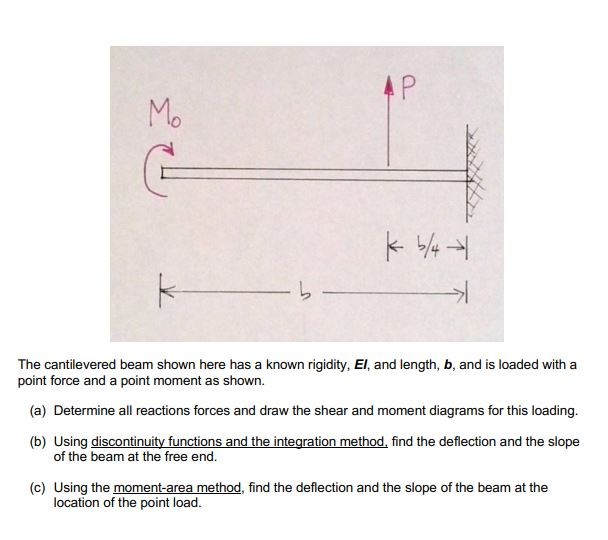AP Mo G kb/4 k > The cantilevered beam shown here has a known rigidity, El, and length, b, and is loaded with a point force and a point moment as shown. (a) Determine all reactions forces and draw the shear and moment diagrams for this loading. (b) Using discontinuity functions and the integration method, find the deflection and the slope of the beam at the free end. (c) Using the moment-area method, find the deflection and the slope of...

• ### answers in terms of P,b and M AP Mo kb/4 k - The cantilevered beam shown...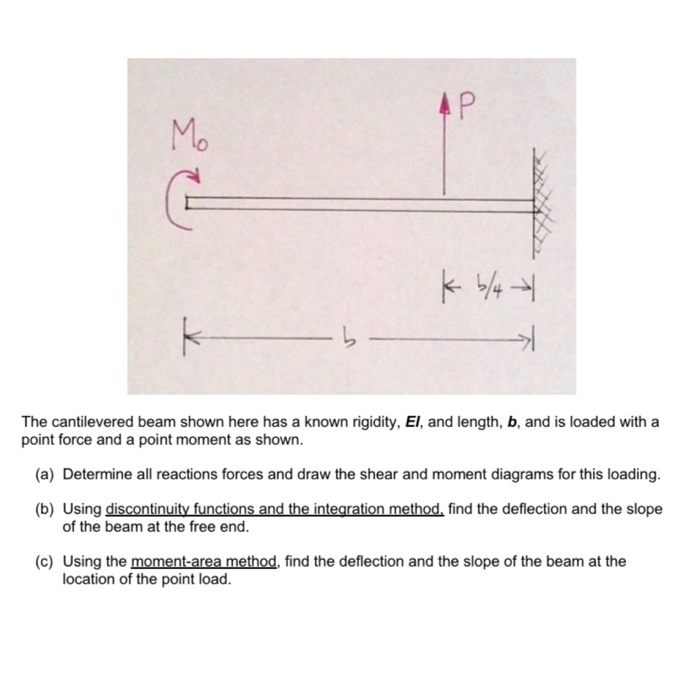answers in terms of P,b and M AP Mo kb/4 k - The cantilevered beam shown here has a known rigidity, El, and length, b, and is loaded with a point force and a point moment as shown. (a) Determine all reactions forces and draw the shear and moment diagrams for this loading. (b) Using discontinuity functions and the integration method, find the deflection and the slope of the beam at the free end. (c) Using the moment-area method, find...

• ### 2. A beam with a uniform flexural rigidity, EI, is loaded by a triangular distributed load,...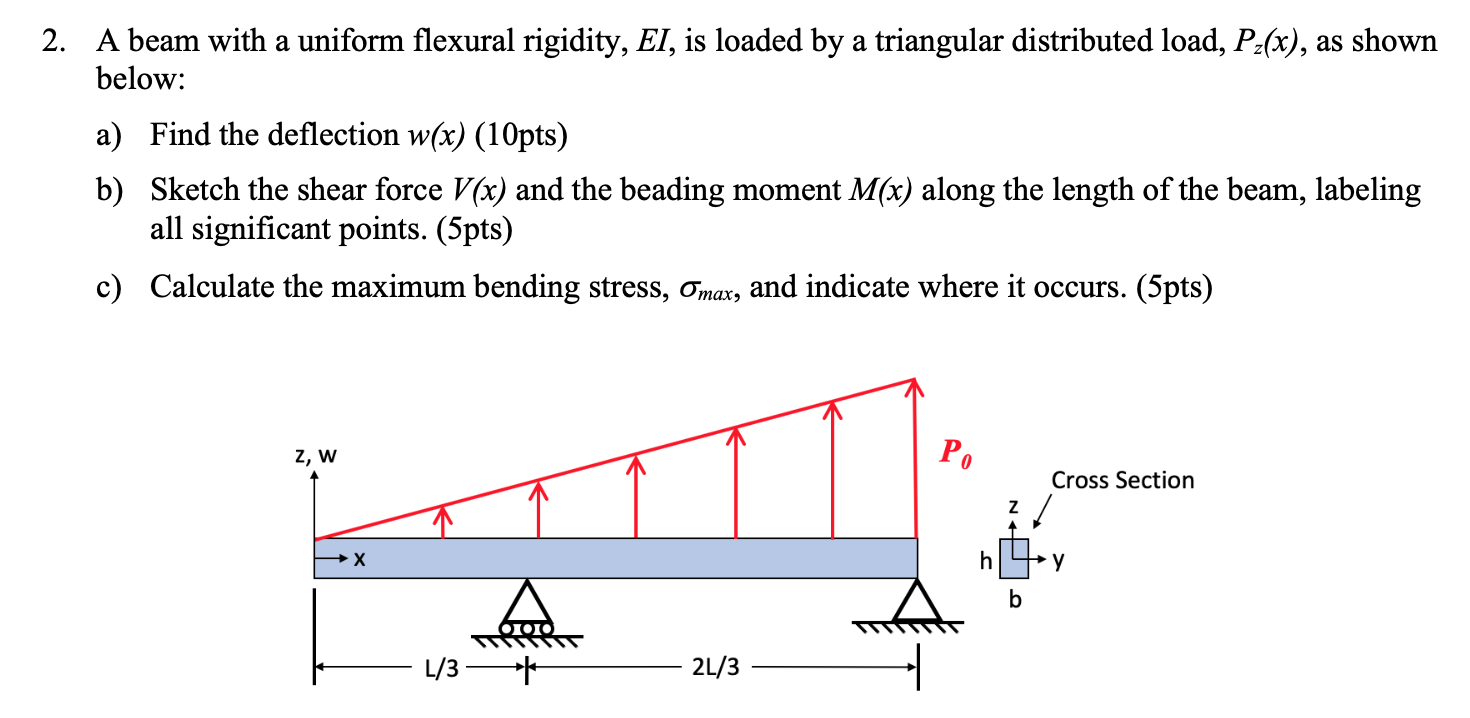2. A beam with a uniform flexural rigidity, EI, is loaded by a triangular distributed load, Pz(x), as shown below: a) Find the deflection w(x) (10pts) b) Sketch the shear force V(x) and the beading moment M(x) along the length of the beam, labeling all significant points. (5pts) c) Calculate the maximum bending stress, Omax, and indicate where it occurs. (5pts) z, W Cross Section - 1/3 — * - 2/3 —

• ### For the loading shown in the below figure, knowing that wo 2 kN/m, the length of the beam is L 2 ...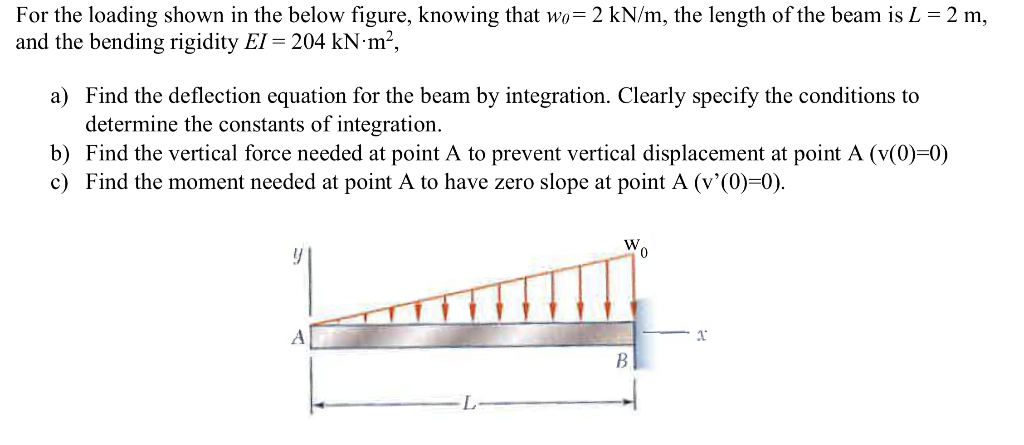For the loading shown in the below figure, knowing that wo 2 kN/m, the length of the beam is L 2 m, and the bending rigidity EI-204 kN-m2, a) Find the deflection equation for the beam by integration. Clearly specify the conditions to determine the constants of integration b) Find the vertical force needed at point A to prevent vertical displacement at point A (v(0)-0) c) Find the moment needed at point A to have zero slope at point A...

• ### PLEASE PROVIDE THE SLOPE AND DEFELCTION DIAGRAM!!!!!!!!!!!!!! 7) A beam is supported and loaded as shown...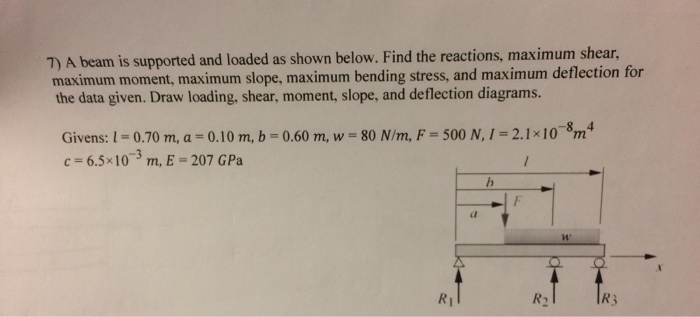PLEASE PROVIDE THE SLOPE AND DEFELCTION DIAGRAM!!!!!!!!!!!!!! 7) A beam is supported and loaded as shown below. Find the reactions, maximum shear, maximum moment, maximum slope, maximum bending stress, and maximum deflection for the data given. Draw loading, shear, moment, slope, and deflection diagrams. -8 4 Givens: 1 0.70 m, a = 0.10 m, b 0.60 m, w 80 N/m, F = 500 N,1 = 2.1x10m c 6.5x10 m, E = 207 GPa / R3 R1 R2 7) A beam...

• ### 7) A beam is supported and loaded as shown below. Find the reactions, maximum shear, maximum...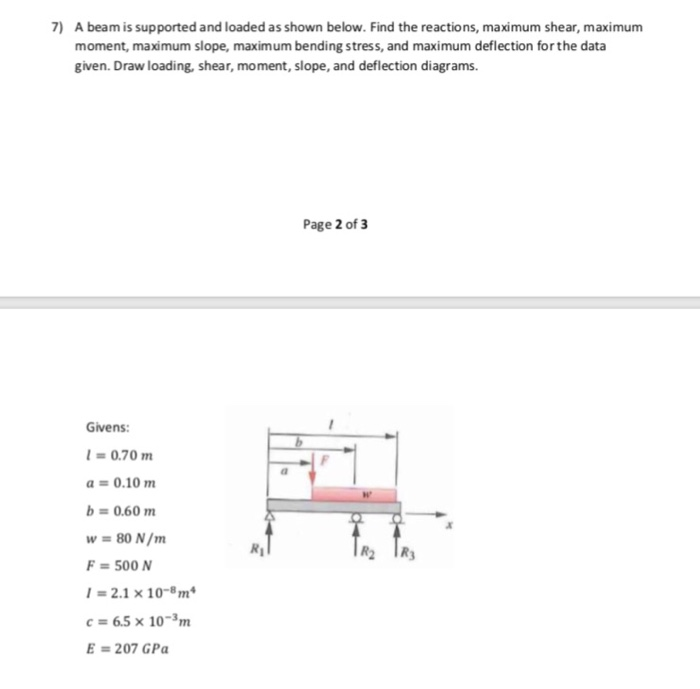7) A beam is supported and loaded as shown below. Find the reactions, maximum shear, maximum moment, maximum slope, maximum bending stress, and maximum deflection for the data given. Draw loading, shear, moment, slope, and deflection diagrams. Page 2 of 3 Givens: 0.70 m a 0.10 m b 0.60 m w 80 N/m F 500 N 2.1 x 10- m c 6.5 x 10-3m E=207 GPa 7) A beam is supported and loaded as shown below. Find the reactions, maximum...

• ### Problem 9.4 The W10x15 cantilevered beam is made of A-36 steel and is subjected to the...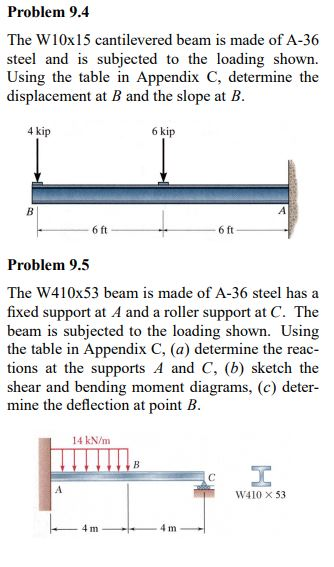Problem 9.4 The W10x15 cantilevered beam is made of A-36 steel and is subjected to the loading shown. Using the table in Appendix C, determine the displacement at B and the slope at B. 4 kip 6 kip В 6 ft 6 ft Problem 9.5 The W410x53 beam is made of A-36 steel has a fixed support at A and a roller support at C. The beam is subjected to the loading shown. Using the table in Appendix C, (a)...